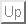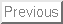Next: Introduction

# The fussy language: Implementation of an automatic error propagation algorithm

S. Bhatnagar

National Radio Astronomy Observatory

Nov. 2003

### Abstract:

Formal propagation of random errors in a mathematical expression follow a precise prescription based on calculus. This requires the computation of the variation of the function with respect to each of the independent variables used to construct the function. These variations are added in quadrature to compute the final numerical error. For complicated expressions, computation of all the partial derivatives is often cumbersome and hence error prone.

The fussy1scripting language, described here, implements an algorithm for automatic propagation of random measurement errors in an arbitrary mathematical expression. It is internally implemented as a virtual machine for efficient runtime performance and can be used as an interpreter by the user. A simple C binding to the interpreter is also provided. Mathematical expressions can be implemented as a collection of sub-expressions, as sub-program units (functions or procedures) or as single atomic expressions. Errors are correctly propagated when a complex expression is broken up into smaller sub-expressions. Sub-expressions are assigned to temporary variables which can then be used to write the final expression. These temporary variables are not independent variables and the information about their dependence on other constituent independent variables is preserved and used on-the-fly in error propagation.

The scripting syntax of fussy is similar to that of C. It is therefore easy to use with minimal learning and can be used in every day scientific work. Most other related work found in the literature is in the form of libraries for automatic differentiation. Only two tools appear to have used it for automatic error propagation. Use of these libraries and tools require sophisticated programing and are targeted more for programmers than for regular every day scientific use. Also, such libraries and tools are difficult to use for correct error propagation in expressions composed of sub-expressions.Next: Introduction
Sanjay Bhatnagar 2011-05-28